The Universe: Notion of Motion

The universe is not static. It cannot exist other than in a permanent state of dynamic equilibrium. Thus, all the free objects, of which it is composed, are in continual motion relative to each other. Motion is vital to its being. If it were not in motion, there would be no universe. [Português]On a clear tropical night, way out in the countryside far from the city lights, I love to stare up at the sky. I see, at various times, the Milky Way, the Southern Cross and Orion with Betelgeuse at its corner. All seems maj­estic, peaceful and still. But in reality, all the stars are in constant mo­tion relative to each other. Betelgeuse is so large that, if it were where the sun is, it would engulf all the in­ner planets within its writhing surface. Even the size and shape of the giant nebula on the right, known as the Hand of God, is con­stantly changing. Galaxies rotate and trans­late relative to each other, as do also mole­cu­les and atoms.

Notwithstanding, motion, within the context of the universe, is not quite the same as it is humanly perceived in everyday life here on the surface of the Earth. The hu­man perception of motion has developed within an environment which is funda­mentally different from universal space. The terrestrial biosphere is the one and only all-pervading frame of reference for the human experience of motion. Conse­quently, it is the base-line of "stationariness" from which the human mind quanti­fies its notion of motion. Because, to us, it is so all-encompassing, it gives us the sense that motion has an absolute measure. After all, most of the things we see — such as mountains, lakes, trees and buildings — always stay where they are. Even things that move — such as people, cows, balls and cars — generally spend more time at rest than they do on the move.As a child, I became familiar with many different kinds of things that move, which I mostly encountered in and around the home of my grandparents. However, I think that the very first thing, which I ment­ally abstracted as an independent moving object, was a soft ball with which I played in my grandparents' garden.

The main reason I was able to mentally abstract my ball as an independent moving object was that I could clearly see its motion relative to me and the rest of the world. When I threw my ball, I perceived that its motion was very complex. It obvi­ously did not go in a straight line. Nevertheless, my neural networks seemed well able to rationalise the ball's motion, thus making it appear quite normal to me. Even when I rolled my ball across the lawn, its motion was still not simple. Its friction with the grass caused it to decelerate non-linearly. But this motion too became rational­ised within my mind as another normal kind of motion.

Many years later, when I began to study mathematics and physics at school, my curiosity was fired into analysing the more fundamental aspects of the motions I had observed with my childhood ball. I had reached an age at which my mind be­came able to create abstract situations in which the friction of the air and grass — and even the effects of gravity — were removed. I became able to perceive the notion of uncontaminated velocity.

From within such abstract situations — or thought experiments — I could clearly see that an unmolested object either stays where it is, relative to me, or changes its position with respect to me at a constant rate. It is either stationary relative to me or it is moving with constant velocity towards me or away from me. The relative as­pect of velocity persuades me that the stationary condition is simply a special case of relative velocity. It is merely zero relative velocity.

It was not until a long time afterwards that I perceived that if the unmolested object I am observing is moving along a path which is not directly in line with me, it will not be moving at a constant velocity relative to me. It will always be — to a greater or lesser extent — in a state of acceleration or deceleration relative to me. The great significance of this is that the acceleration and deceleration involved are not provoked by any external force.

Passive Acceleration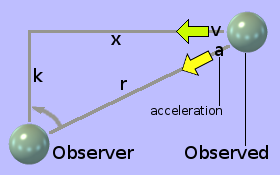The diagram on the left shows an object trav­elling through empty space along the x-axis at a velocity v within the frame of reference of an observer. The object will pass closest to the observer at a distance k. The observer sees the object coming to­wards him as if directly along the hypoten­use track r. To the observer, the object app­ears to be moving towards him at an ever-decreasing speed.In the triangle shown above, k is constant, while x and r both dim­inish with time. Since k is the distance of closest approach, the triangle is right-angled. Its sides x, r and k are therefore related by Pythagoras's squares rule, as shown on the right. Since k is con­stant, the rate at which r changes with x can be calculated by different­iating Pythagoras's equation as shown on the right.Using the differentiation chain rule, we can now find how the dist­ance r varies over time. The differential of x with respect to time is the vel­ocity v of the object along the x-axis. This we deem to be constant because we decreed that the object travel along its path at constant velocity. No force is acting upon it.The task now is to find if, and if so then by how much, the object is accelerating, along the path r, towards the obser­ver. Using the standard rule to calculate the sec­ond deriva­tive, we find that the second term is zero because we de­creed that the velocity, v, of the object along the x-axis be constant.To complete the calculation of the acceleration, a, along r, we must evaluate the second derivative of r with respect to x. This is effectively the derivative of the first derivative of r with res­pect to x, which we found to be x/r. Rather than deal with the reciprocal of r, I prefer to substitute a new variable u = 1/r. Then the problem becomes to differentiate the product of the two related variables x and u.

On the left, I have used the product rule to find the second de­rivative. I have put in each laborious step in the process rather than jump several steps at once so that nobody should find any trouble following it. My hope is that I have actually got it right, since it is many decades since I was actively doing this kind of mathematics.

In the result, k2/r3, k2 is a constant. So the rate of change of the rate of change of r with respect to x is inversely pro­portional to r3. To get the acceleration, a, along the direct line between the observed and the observer, we simply need to multiply this re­sult by v2.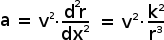Since v2 is also a constant, the acceleration, a, of the object towards the observer along r is still inversely proportional to r3. Thus it is extremely non-linear.

It is clear that differentiating further to find the rate of change of acceleration, the rate of change of the rate of change of acceleration and so on, will involve even higher inverse powers of r. Each successive differential will have the opposite sign from its predecessor and will contribute less and less to the nature of the relative motion between the object and its observer. The true final form of the acceleration of the object towards its observer will be the amalgamation of an infinite series of differentials.

Notwithstanding, the clear result is that two bodies travelling through space at diff­erent constant velocities (with no external force or gravitational distortion acting upon either) each experiences the other first decelerating towards and then accel­erating away from it. It is as if a thrower on the ground throws a ball to a catcher up a tree and the ball rises towards the catcher and then falls back down again before the catcher can grab it. Note, however, that the formula for the acceleration under gravity is somewhat different.

A good sense of this kind of motion can be got from observing a water, cellphone or microwave tower some distance from the track, as you pass by it in a high-speed train or by watching the control tower at an airport from an aircraft as it is landing.

We assume that the above scenario takes place in an empty universe with no stars to give any sense of a more universal frame of reference. The only objects in this universe are the observer and the observed. Without a starry background, the observed simply appears to approach the observer at velocity v, which reduces at an ever-increasing rate. It then stops approaching when it reaches distance k and then accelerates away at an ever-reducing rate of acceleration but at a velocity which keeps on increasing until it tends towards its upper limit v.

This behaviour would appear consistent with the proposition that the observer and the observed are exerting upon each other a repulsive force which is approximately inversely proportional to the cube of their separation.

Interjection: If the passing objects were to repel each other under an inverse square law, the way passing electrons would do, they would not continue on their respective "straight" trajectories. Each would perturb the other, away from its original course, along a hyperbolic trajectory.

Yet, for neutral passing objects, no force [inverse square or otherwise] is involved: only relative velocities and angles. The repulsive force is an illusion of relative mo­tion. Could other repulsive forces — such as some of those in the sub-atomic world — also be ultimately resolved as illusions of relative motion?

There is one little twist to this scenario. For the observed to appear to travel direct­ly towards the observer, stop, then move away again, the observer must rotate his head (or direction of view). Furthermore, the rate at which he must move his head, to keep the observed object directly in line, is non-linear. He must apply an angular acceleration and then an angular deceleration. And that requires the application of a specifically varying external force in the form of a torque. Perhaps this apparently forceless acceleration is somehow related to a rotational torque. The apparent force of repulsion thus only appears within the frame of reference of an observer who is undergoing the appropriate rotational acceleration.

Notwithstanding, if the observer were to keep looking ahead in the direction along k, the observed object would appear to approach him, swing past in front of him then veer away again along, what would appear to him as, a hyperbola-like path — somewhat similar in nature to the path taken by a repelled charged particle.

For the most part, therefore, the relative velocity between an observer and an ob­served, within a forceless scenario, is not constant. There does exist, however, one direction in space in which the relative velocity between an observer and the obser­ved is constant. That is when the observer and the observed are on a direct collis­ion course. And this unique situation does not require the observer to rotate his head in order to keep the observed object in the centre of his view.

In summary, therefore, the presence of non-linear relative acceleration, between two objects in free space, necessitates neither the application of a directed external force nor the presence of any mysterious 'action at a distance' or 'distortion in the fabric of space-time'. It is entirely a consequence of simple Euclidean geometry.

In Free-FallSuppose I throw a mass, such as the green sphere shown on the left, out of an aircraft in flight. It will accelerate to­wards the ground at 9·80665 metres per second per sec­ond. Yet no external force is acting upon it. [Any external force must — by definition — be applied at some part of a body's outer surface. The force is then distributed to every part of the body by stresses and strains, set up by the force, within the material structure of the body itself.] Thus we have another situation in which a mass can be acceler­ating without an external force acting upon it. Notwithstan­ding, if we work backwards from acceleration, using Isaac Newton's observation that force = mass × acceler­ation for a directed external force acting upon a mass in free space, we arrive at a value which, to our Earth-bound perception, resembles some kind of phantasmagorical force acting upon the mass.

However, unlike a real force acting on a mass in free space, this ghostly force of free-fall does not act upon the external surface of a free-falling body. Neither does it thereby set up any internal stresses and strains within the material structure of the body to distribute itself to all parts of the body's mass. It is as if this ghostly force were infinitely divided up in order for it to act directly and independently on every last molecule, atom, primary-particle, string or whatever makes up the ess­ence of the mass. So, again, it is not the same kind of thing as a real external force.

Expanding the scope of my view, I see that the notion of free-fall is, in the strictest sense, two masses falling towards each other: the one being the little mass I threw out of the aircraft and the other being the Earth. Furthermore, the rate at which they accelerate towards each other is not the neat and linear 9·80665 metres per second per second: it is a complicated kind of acceleration which is extremely non-linear.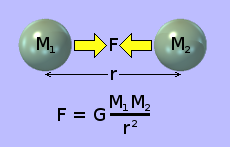The apparent force, which seems to pull two objects in free space towards each other is shown in the illu­stration on the right. This apparent force gets enor­mously stronger as the two objects get closer togeth­er, as shown by the formula at the bottom of the dia­gram. This fictitious force appears to accelerate the objects towards each other at a rate that is continu­ally increasing.

Please note: this formula is derived from direct observation and measurement, and the constant 'G' is extremely small: 6·67408 × 10−11.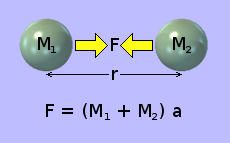If this force were a real externally applied force (which it isn't), it would accelerate the two objects towards each other according to the formula sho­wn on the left. Scientists have taken the dubious liberty of regarding the phantasmagorical force of attraction between the two objects, and the real external force required to produce the same ac­celeration, to be equivalent.

This formula is also derived from direct observation and measurement.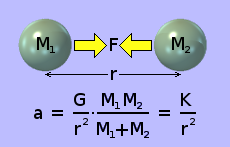They thereby arrive at a formula, shown on the right, for calculating the acceleration produced by so-call­ed gravitational attraction. Assuming the two masses involved remain constant, the formula reduces to ac­celeration, a = K ÷ r2, where K is a constant. From an engineering point of view, the scientists are vindica­ted. The formula works! And to an amazing accuracy.

Notwithstanding, from a conceptual point of view, equating this fictitious force of attraction with the real force that would produce the same acceleration, remains problematic. When an object is in free-fall, there is no tangible force acting upon it. The only situation in which gravity exerts a tangible force is when an object is rest­ing on the ground. So what is really happening? What is the conceptual truth?

The acceleration of the two masses towards each other is extremely non-linear. It is inversely proportional to the square of the distance between the masses. It is interesting to recall, however, that the acceleration of two objects passing each other on a non-collision course in free space is inversely proportional to the cube of the distance between the objects. So, horrendous non-linear acceleration is no big deal. It does not necessarily mean that some mysterious force is involved.

The presence of acceleration and the absence of a tangible external force suggests that, in the process of falling towards each other, the two masses are in a similar state as two free objects on a non-collision course in free space. In fact, if a real external force is applied to an object in free-fall, it will — independently of its accel­eration under free-fall — accelerate in exactly the same way as does an object in free space far away from any planet. Vectorially, its motion under the influence of the real force is simply superimposed on top of its acceleration under free-fall.

There is, however, a crucial difference. The real external force has to overcome the inherent inertia of the object in order to accelerate it. The phantasmagorical force of gravity does not. When an object is in free-fall, it is exhibiting no inertial react­ion. If it is thus accelerating, and if no real force is required to maintain that accel­eration, then, it would seem that, in this situation, the object has no inertia. It is still a material object, which we presume must therefore have mass. But it has no in­ertia. This again suggests to me that perhaps, in reality, inertia is not a property of matter, but of space.

In Orbit

Free-fall — when two objects fall towards each other on a direct collision course — is really only one special case of what is called a gravitational encounter.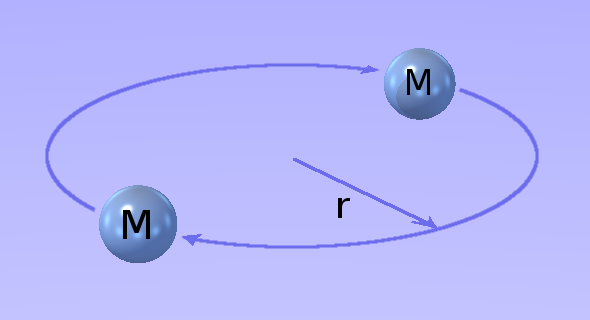The general case is where the two objects approach from opposite infinities, pass by each other on non-collision paths, then dep­art from each other towards two other oppo­s­ite infinities. The paths they follow are hyp­er­bolic. Other special cases are where their paths are parabolic, elliptical or circular. The case of two objects in cir­cular orbit is shown on the right.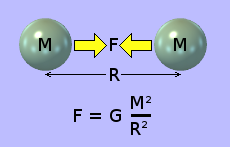According to conventional science, the two objects in orbit around each other are attracting each other gravitationally with a force F, which is given by the formula shown on the left.

Please note that, in this formula, R (capital) is the distance separating the two objects, which is twice the radius of the orbit, r (small).

Thus, the fictitious force of attraction, F = G × (M ÷ (2 × r))² = GM²/4r².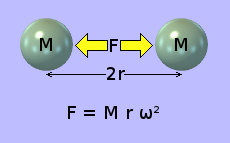In a stable circular orbit, this fictitious force of attr­action is said to be balanced out by an equal and opposite centrifugal force, F, which is given by the formula shown on the right. F is the force that pulls a weight outwards when you swing it round on a str­ing. In this formula, ω is the angular velocity (in rad­ians per second) with which the objects are revolving round their common centre.

The above formula is essentially the same as Isaac Newton's observation that force = mass × acceleration, but applied to motion in a circle instead of a straight line. Acceleration is rate of change of velocity. Velocity is speed in a straight line. It requ­ires force to change the direction in which an object is travelling. Therefore when an object moves in a circle, its velocity is constantly changing [it is accelerating], even though the object's path-speed — and hence, its angular velocity — remain constant.

If these fictitious attractive and centrifugal forces are equal and opposite, then:

ω² ω² M × r × ω² = G × M × M ÷ (4 × r × r) = G × M ÷ (4 × r × r × r) = G × M ÷ (4 × r³) = GM/4r³

Thus the orbital angular velocity ω of the two objects around the common centre for a stable circular orbit is given by the formula: ω = √(GM/4r³) radians per sec­ond.

Here again, as with linear motion, neither object is being subjected to any directed external force. If it were a sentient observer, it would feel nothing. No external agency is pushing or prodding it in any direction. There is no external force, applied at any point on its surface, being distributed to all its parts via stresses and strains within its material structure.

And here again, since the objects are clearly accelerating without the presence of any external forces to accelerate them, it appears that the two bodies orbiting around each other have no inertia. No force: no inertial reaction. But nonetheless, they are in a state of constant acceleration.

Motion Without Force

Unfortunately, a human upbringing, within the biosphere of Planet Earth, does not help us when trying to understand motion in free space. Within our earth-bound experience, nothing moves unless it is pushed. In other words, without an external force, things simply stay put. And it is upon this all-pervading experience that our concepts of movement are naturally and subconsciously based.

But out in space it doesn't work like that. In free space, any object, which is not be­ing acted on by a directed external force, is at rest. But at rest with respect to what? The essence — or substance — of space! Such an object may be at rest with re­spect to certain other objects. It may be travelling at incredible velocities relative to others. It may even be undergoing extremely non-linear accelerations relative to yet others. But relative to the essence or substance of space, it is at rest. It is not moving. And the same is true of all other objects not being acted on by directed external forces.

The above notion of motion — or rather the absence of such motion — relative to the essence or substance of space is quite alien to everyday human experience. How­ever, I think that this is not so much due to our terrestrial notion of motion but rather to our terrestrial conception of space. Our basic notion of space, here on the Earth, is that of distance: the static separations between different locations.The basic concept out in free space, however, is force. A material object clearly exists in 3 dimen­sional x,y,z space. Notwithstanding, the essence or substance of that space is oblivious to what­ever passive state of motion the object may have with respect to other objects. It only reacts — and thereby reveals its existence — when a directed force is applied to the object. Thus, it can be said that the essence or substance of space exists only in a force-type f,g,h frame of refer­ence, as de­picted on the left. And within this f,g,h frame of reference, the essence or sub­stance of space app­ears to have the nat­ure of a static viscous fluid.

Consequently, if I am in free space, far from the sight or influence of any other obj­ect and with no external force acting upon me, I am at rest with respect to the ess­ence or substance of space. Equally consequently, if I am in accelerating free-fall towards a planet, again with no external force acting upon me, I am also at rest with respect to the essence or substance of space.

Space has size. Therefore it cannot be nothing. Therefore it must have essence or substance of some kind. I call the essence or substance of space the æther. I ima­gine the æther as having the nature of a fluid. Space contains objects. These ob­jects have very complex relative motions. Yet each one — upon which no directed ex­ternal force is acting — is at rest with respect to the æther.

To reconcile the notion of objects being in motion relative to each other in x,y,z space with the notion of each one being at rest with respect to the æther, the æther itself must be in a continual state of motion. It must, at different times in different places, be expanding, contracting, warping and buckling.

Alternatively, one could surmise that it is the æther that is stationary and homogeneous and that it is x,y,z space that is expanding, contracting, warping and buckling.

And all this takes place un-driven by external forces. Complex passive motion is the ground state of the universe.

© 26 April 2016 Robert John Morton | PREV | NEXT